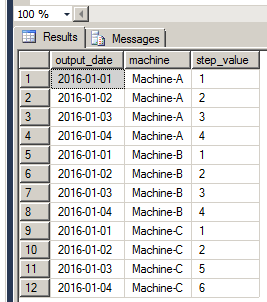Select PageThis advanced SQL exercise is part of the book: Advanced SQL – Practical Techniques and Use-Cases, which includes 100 day-to-day SQL challenges (and solutions), need-to-know advanced features, and key concepts.

A semiconductor fabrication plant has 3 operating machines. At the end of each day, each of these machines generates a numeric output that represents the task it completed on that day (step_value). On the following day, the task equals yesterday’s value + 1. For example:

• On January 1st Machine-A generated the numeric value 1, on the following day the machine generated the value 2, the day after: value 3, and so on (normal operation).
• On January 1st Machine-B generated the numeric value 1, on the following day the machine generated the value 2, the day after: value 3 ,and so on (normal operation).
• On January 1st Machine-C generated the numeric value 1, on the following day the machine generated the value 2, the day after: value 5 instead of 3 (an error has occurred).
```
CREATE TABLE machines_output
(output_date date,
machine varchar(25),
step_value int)
GO

```